# High School Physics : Electricity

## Example Questions

### Example Question #1 : Electricity

What is the maximum power consumption of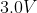portable CD player that draw a maximum of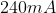of current?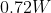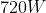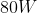Explanation:

The power in a circuit is equal to the current times the voltage.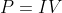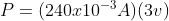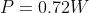### Example Question #1 : Electricity

The total amount of charge that passes through a wire’s full cross section at any point per unit of time is referred to as

Wattage

Electric potential

Current

Voltage

Current

Explanation:

Current is defined as the amount of charge that passes through a specific area of a wire in a specific interval of time.  It is measured in Amps which are Coulombs per second.

### Example Question #4 : Electricity

If the resistance in a constant voltage circuit is doubled, the power dissipated by that circuit

Decrease to one fourth its original value

Decrease to one half its original value

Increase by a factor of four

Increase by a factor of two

Decrease to one half its original value

Explanation:

Decrease to one half its original value

The equation for Power isAccording to Ohm’s Law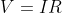We can rearrange this equation for current as this is what is changing in our circuit.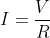We can then substitute this back into the power equation.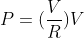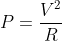If we have the original value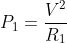and our second value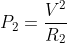We can rearrange both for the voltage and set them equal to each other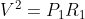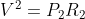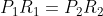So we can substitute this value in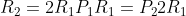And solve for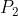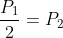Therefore the power would be cut in half.

### Example Question #5 : Electricity

A current of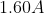flow in a wire.  How many electrons are flowing past any point in the wire per second?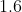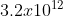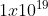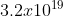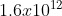Explanation:

The current is a measure of the amount of charge that passes a given point in a certain amount of time.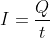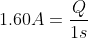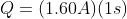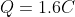To determine how many electrons are passing this point we need to look at the charge of 1 electron and do a conversion.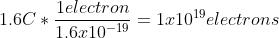### Example Question #1 : Electricity

The direction of conventional current is taken to be the direction that __________________ .

negative charges would flow

positive charges would flow